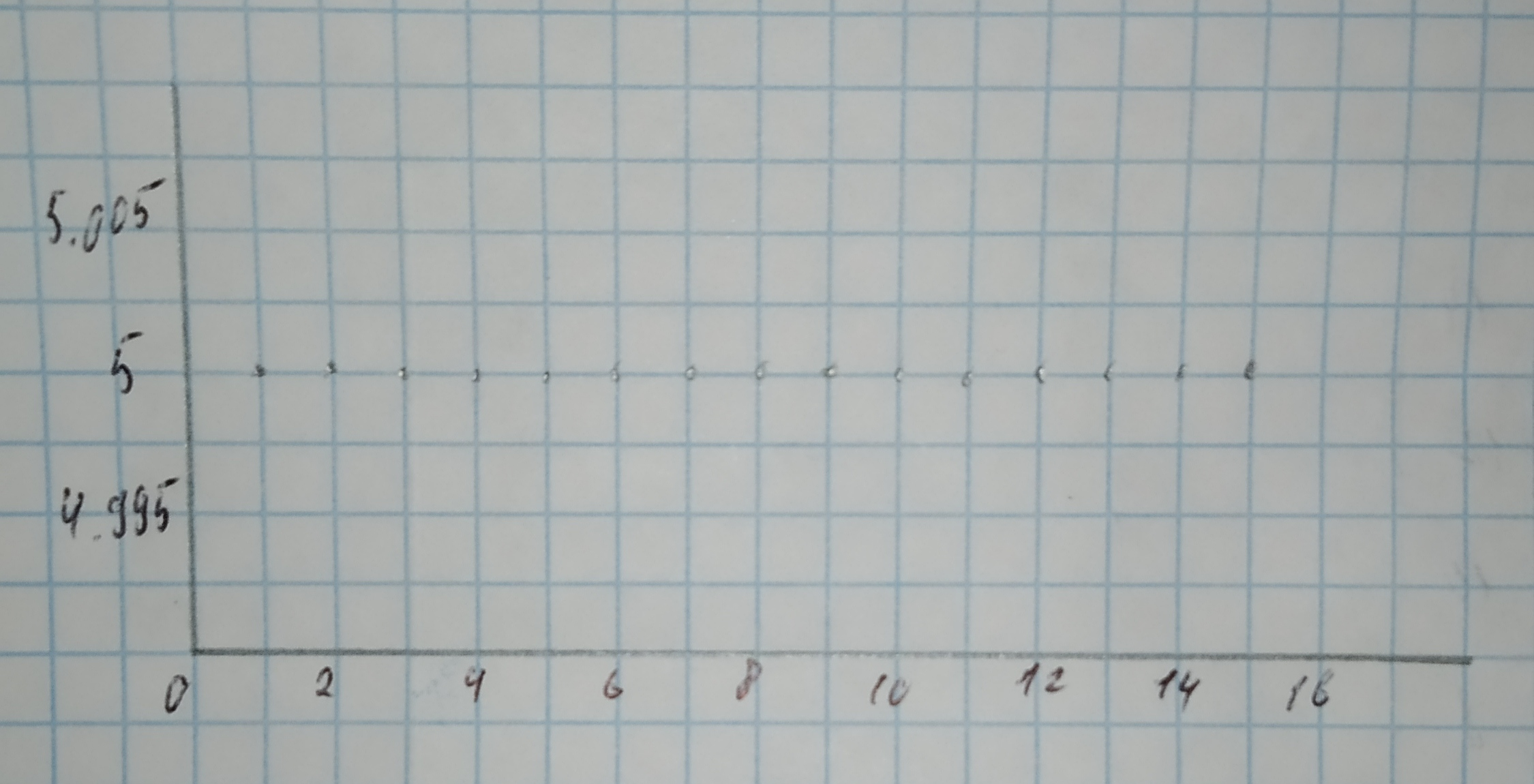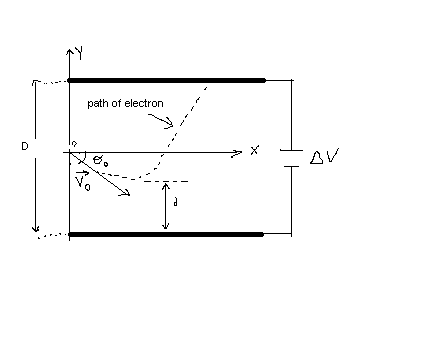Ask question

# Sketch a scatterplot where the association is linear, but the correlation is close to [r = 0].# Sketch a scatterplot where the association is linear, but the correlation is close to [r = 0].

Question
Scatterplotsasked 2021-03-04
Sketch a scatterplot where the association is linear, but the correlation is close to [r = 0].

## Answers (1)2021-03-05
An association is linear when there is no strong curvature present in the scatterplot.
The correlation is close to [r = 0], when the pattern slopes does not slope upwards nor downwards.
More precisely, an association that is linear with a correlation coefficient close to 0 needs to have a pattern that is (nearly) horizontal in the scatterplot.
For example, the following graph then has a correlation close to [r = 0] while also being linear.### Relevant Questionsasked 2020-10-26
Sketch a scatterplot where the association is nonlinear, but the correlation is close to [r = -1].asked 2021-02-19

Make a scatterplot for each set of data. Tell whether the data show a linear association or a nonlinear association.
$$(1,\ 2),\ (7,\ 9.5),\ (4,\ 7),\ (2,\ 4.2),\ (6,\ 8.25),\ (3,\ 5.8),\ (5,\ 8),\ (8,\ 10),\ (0,\ 0)$$asked 2021-05-31
Make a scatterplot for the data.
Height and Weight of Females
Height (in.): 58, 60, 62, 64, 65, 66, 68, 70, 72
Weight (lb): 115, 120, 125, 133, 136, 115, 146, 153, 159asked 2020-11-01
Sketch a scatterplot showing data for which the correlation is [r = -1].asked 2020-12-25
In this exercise, you will use the correlation and regression applet to create scatter plots with 10 points that have a correlation close to 0.7. The lesson here is that many models may have the same correlation. Always compile your data before trusting correlations. (a) Stop after adding the first two points. What is the value of correlation?
(Enter your answer, rounded to four decimal places).
r=?
Why does correlation matter? Two is the minimum number of data points required to calculate the correlation. This value is the default correlation.
Because two points define a line, correlation always matters.
The mean of these two values always has this value.asked 2020-12-15
A researcher investigating the association between two variables collected some data and was surprised when he calculated the correlation.
He had expected to find a fairly strong association, yet the correlation was near 0.
Discouraged, he didn't bother making a scatterplot.
Explain to him how the scatterplot could still reveal the strong association he anticipated.asked 2021-02-25
Use a scatterplot and the linear correlation coefficient r to determinewhether there is a correlation between the two variables.
[x 1 2 2 5 6 y 2 5 4 15 15]asked 2020-11-01
The value of s alone doesn't reveal information about the form of an association. Sketch a scatterplot showing a nonlinear association with a small value of s and a second scatterplot showing a linear association with a large value of s.asked 2020-11-05
Sketch a scatterplot in which the presence of an outlier decreases the observed correlation between the response and explanatory variables. Indicate on your plot which point is the outlier.asked 2021-05-12
An electron is fired at a speed of $$\displaystyle{v}_{{0}}={5.6}\times{10}^{{6}}$$ m/s and at an angle of $$\displaystyle\theta_{{0}}=–{45}^{\circ}$$ between two parallel conductingplates that are D=2.0 mm apart, as in Figure. Ifthe potential difference between the plates is $$\displaystyle\triangle{V}={100}\ {V}$$, determine (a) how close d the electron will get to the bottom plate and (b) where the electron will strike the top plate....